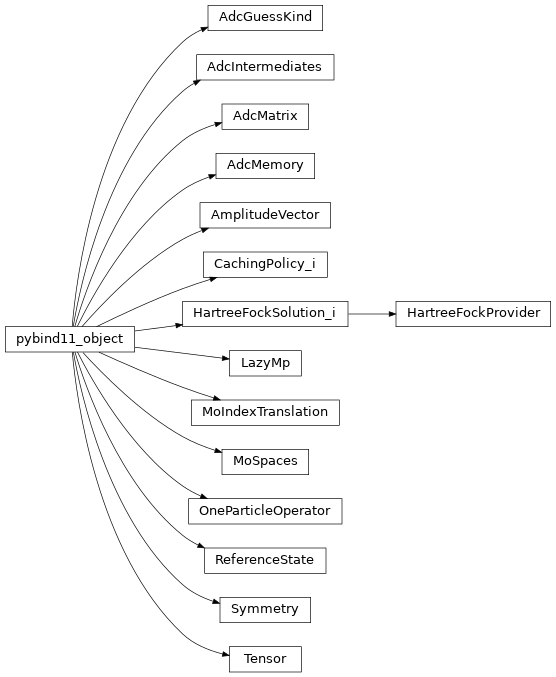The libadcc Python module contains the python bindings of adccore: C++ core library. They are generated directly from the C++ source code using pybind11 and the sources contained in the extension subfolder of the adcc github repository.

It is not recommended to call these functions directly, but instead resort to the higher-level functionality from adcc. See the adcc reference for details.

 `add`(arg0, arg1, arg2) `amplitude_vector_enforce_spin_kind`(arg0, …) Apply the spin symmetrisation required to make the doubles and higher parts of an amplitude vector consist of components for a particular spin kind only. `compute_gs2state_optdm`(arg0, arg1, arg2, arg3) `compute_modified_transition_moments`(arg0, …) `compute_state2state_optdm`(arg0, arg1, arg2, …) `compute_state_diffdm`(arg0, arg1, arg2, arg3) `contract`(arg0, arg1, arg2) `contract_to`(arg0, arg1, arg2, arg3) `divide`(arg0, arg1, arg2) `get_n_threads`() Get the number of running worker threads used by adcc. `get_n_threads_total`() Get the total number of threads (running and sleeping) used by adcc. `guess_symmetries`(arg0, arg1) Return a list of symmetry objects in order to construct the singles / doubles part of the AmplitudeVector. `guess_zero`(arg0, arg1) Return an AmplitudeVector object filled with zeros, but where the symmetry has been properly set up to meet the requirements of the AdcGuessKind object `guesses_from_diagonal`(arg0, arg1, arg2, …) Obtain guesses by inspecting a block of the diagonal of the passed ADC matrix. `make_symmetry_eri`(arg0, arg1) Return the Symmetry object like it would be set up for the passed subspace of the electron-repulsion tensor. `make_symmetry_operator`(arg0, arg1, arg2, arg3) Return the Symmetry object for an orbital subspace block of a one-particle operator `make_symmetry_operator_basis`(arg0, arg1, arg2) Return the symmetry object for an operator in the AO basis. `make_symmetry_orbital_coefficients`(arg0, …) Return the Symmetry object like it would be set up for the passed subspace of the orbital coefficients tensor. `make_symmetry_orbital_energies`(arg0, arg1) Return the Symmetry object like it would be set up for the passed subspace of the orbital energies tensor. `multiply`(arg0, arg1, arg2) `set_n_threads`(arg0) Set the number of running worker threads used by adcc `set_n_threads_total`(arg0) Set the total number of threads (running and sleeping) used by adcc. `subtract`(arg0, arg1, arg2)
 `AdcGuessKind` Class which collects information about the kind of guess vectors to be constructed. `AdcIntermediates` Class holding the computed ADC intermediates. `AdcMatrix` Class representing the AdcMatrix in various variants. `AdcMemory` Class controlling the memory allocations for adcc ADC calculations. `AmplitudeVector` Class representing an AmplitudeVector. `CachingPolicy_i` Should a particular tensor given by a label, its space string and the string of the spaces involved in the most expensive contraction be stored. `HartreeFockProvider` Abstract class defining the interface for passing data from the host program to adcc. `HartreeFockSolution_i` Interface class representing the data expected in adcc from an interfacing HF / SCF program. `LazyMp` Class representing information about the Mo/ller-Plesset results from ADCman. `MoIndexTranslation` Helper object to extract information from indices into orbitals subspaces and to map them between different indexing conventions (full MO space, MO subspaces, indexing convention in the HF Provider / SCF host program, … `MoSpaces` Class setting up the molecular orbital index spaces and subspaces and exposing information about them. `OneParticleOperator` Class representing a one-particle operator. `ReferenceState` Class representing information about the reference state for adcc. `Symmetry` Container for Tensor symmetry information `Tensor` Class representing the Tensor objects used for computations in adcc